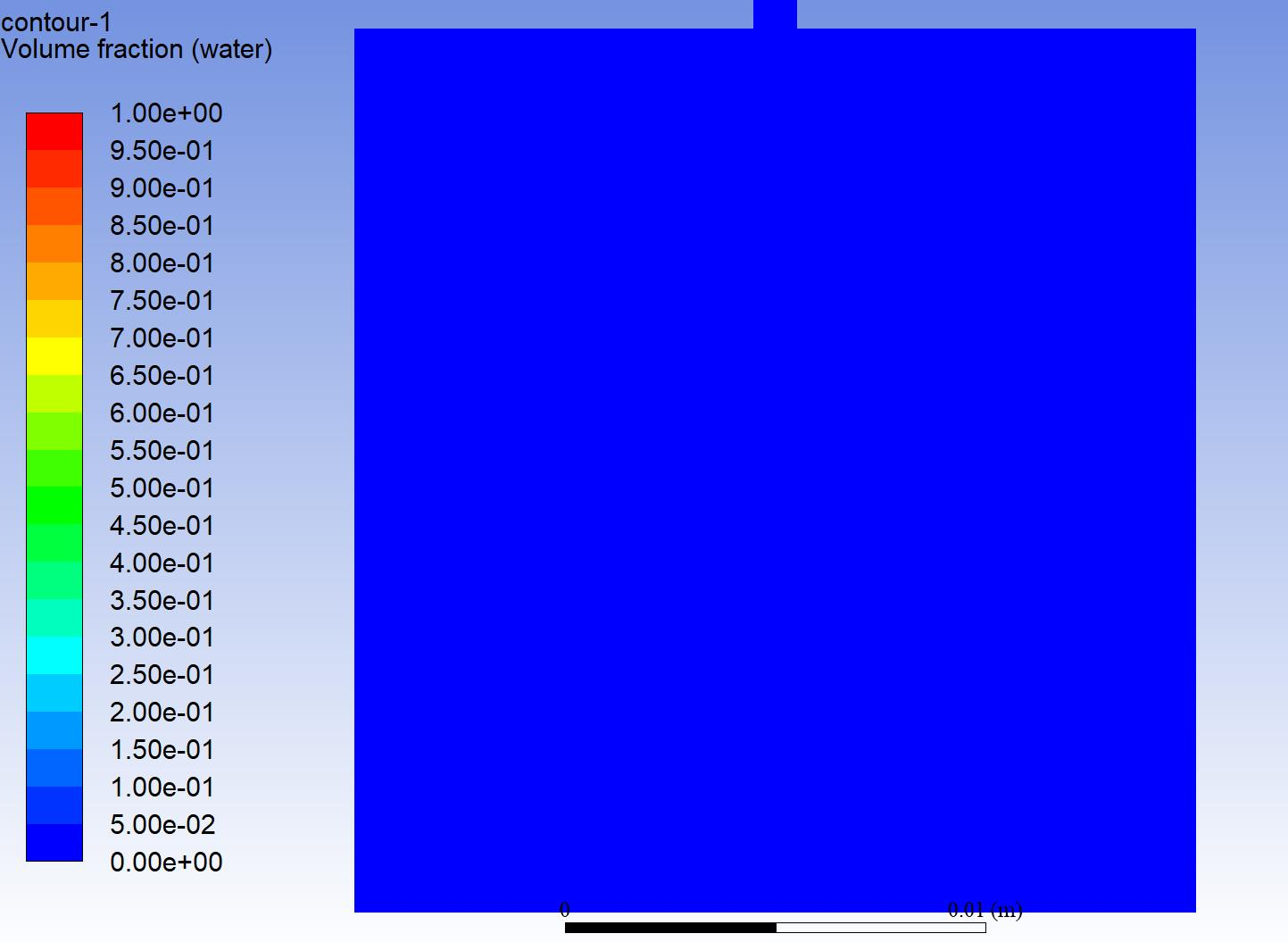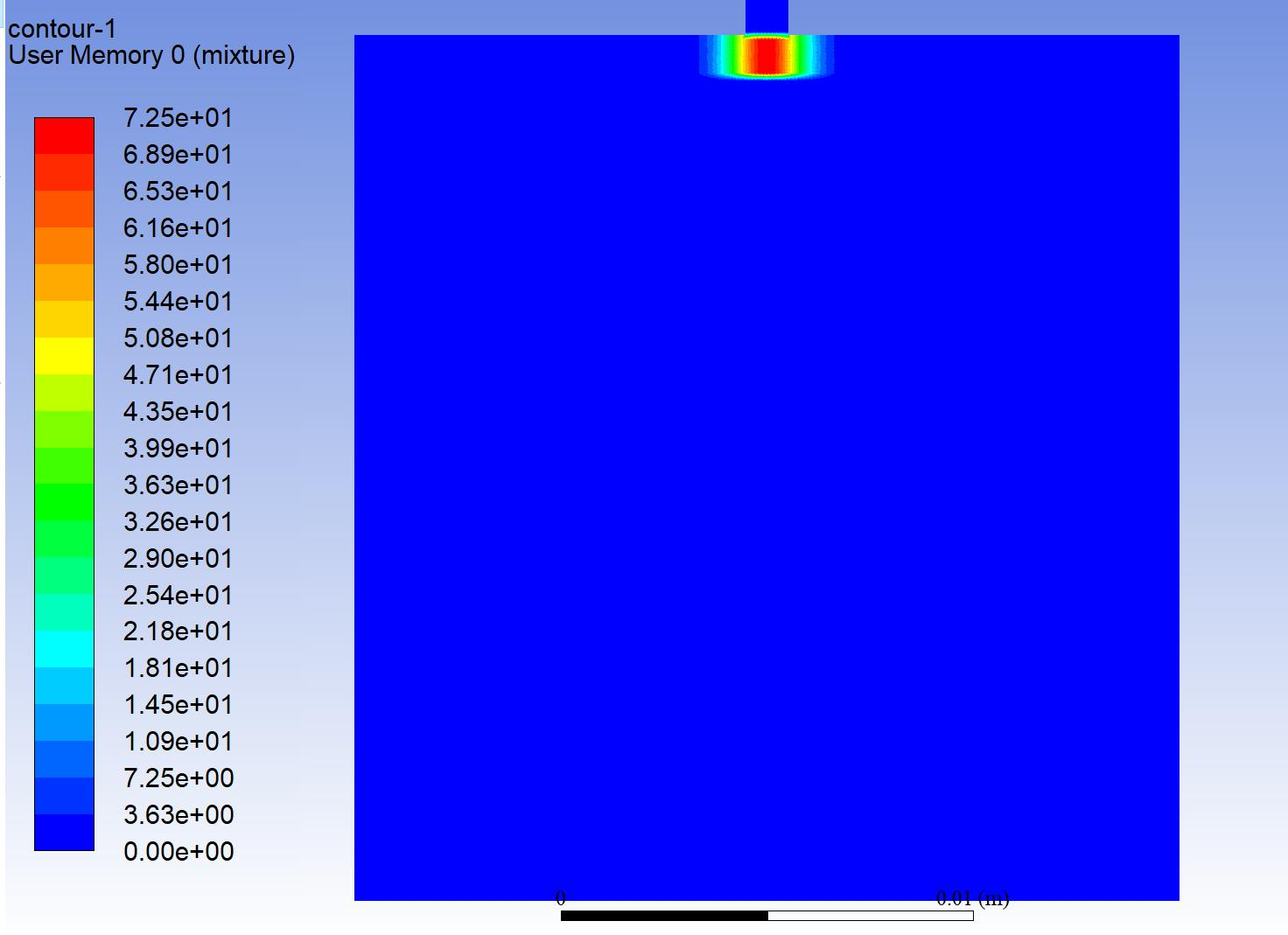## Fluids

•BillT
Subscriber

Hi,

I am modeling multiphase flow with two phases (water and air). I created a mass source term for my water phase. I also activated gravity in the negative y-direction. When my simulation is finished i cannot see any volume fraction of my water. Any suggestions?

Thank you in advance!Mass source:•DrAmine
Ansys Employee

You need to provide secondary fluxes to. But what do you want to do? Can you share your contour plot of VOF direct after applying the source term or just add a report of volume average of VOF?

•BillT
Subscriber

After Initialization it looks the same as above (no water volume fraction). But I customized my mass source term and set the volume fraction of each cell equal to my source. But shouldnt this happen automatically because i am defining a mass source?

FYI, my source term:

#include "udf.h"
#define j 20.0 /* Volumenstromdichte Flüssigphase i m^3/(m^2 h)*/
#define D 0.02 /*Schichtungsdurchmesser oder Kolonnendurchmesser in m*/
#define Pi 3.141592653
DEFINE_SOURCE(masswater, c, t, dS, eqn)
{
real x[ND_ND];
real source, sum, rad;
C_CENTROID(x, c, t);
sum = ((j*C_R(c, t) / 2)*((D*D)*Pi / 4))/3600;
rad = 23.18 * exp(-1122000 * (x*x));

source = sum * rad;
C_VOF(c, t) = source;
C_UDMI(c, t, 0) = source;
return source;
}

•DrAmine
Ansys Employee
Udmi need a to be defined for mixture thread and not for phase thread. Volume fraction is always normalized even if you provide a tonne per second. Where are you going the UDF? Do you need an UDF? Mass source term has to be per volume.
•BillT
Subscriber

What does it mean that the volume fraction is always normalized?

I want to model a point source for a porous zone. Therefore I want to implement a mass source for my water phase in the particular cell zone.

•DrAmine
Ansys Employee

It means the conservative volume fraction can be larger then one but it is normalized so that it does not exceed 1 or be smaller then 0.